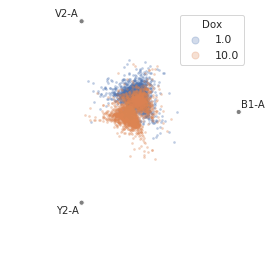class cytoflow.views.radviz.RadvizView[source]

Plots a Radviz plot. Radviz plots project multivariate plots into two dimensions. Good for looking for clusters.

channels

The channels to view

Type: List(Str)
scale

Re-scale the data in the specified channels before plotting. If a channel isn’t specified, assume that the scale is linear.

Type: Dict(Str : {“linear”, “logicle”, “log”})
xfacet, yfacet

Set to one of the conditions in the Experiment, and a new row or column of subplots will be added for every unique value of that condition.

Type: String
huefacet

Set to one of the conditions in the in the Experiment, and a new color will be added to the plot for every unique value of that condition.

Type: String
huescale

How should the color scale for huefacet be scaled?

Type: {‘linear’, ‘log’, ‘logicle’}

Notes

The Radviz plot is based on a method of “dimensional anchors” . The variables are conceived as points equidistant around a unit circle, and each data point connected to each anchor by a spring whose stiffness corresponds to the value of that data point. The location of the data point is the location where springs’ tensions are minimized. Fortunately, there is fast matrix math to do this.

As per , the order of the anchors can make a huge difference. I’ve adapted the code from the R radviz package  to compute the cosine similarity of all possible circular permutations (“necklaces”). For a moderate number of anchors such as is likely to be encountered here, computing them all is completely feasible.

References

  Hoffman P, Grinstein G, Pinkney D. Dimensional anchors: a graphic primitive for multidimensional multivariate information visualizations. Proceedings of the 1999 workshop on new paradigms in information visualization and manipulation in conjunction with the eighth ACM internation conference on Information and knowledge management. 1999 Nov 1 (pp. 9-16). ACM.
  Di Caro L, Frias-Martinez V, Frias-Martinez E. Analyzing the role of dimension arrangement for data visualization in radviz. Advances in Knowledge Discovery and Data Mining. 2010:125-32.

Examples

Make a little data set.

>>> import cytoflow as flow
>>> import_op = flow.ImportOp()
>>> import_op.tubes = [flow.Tube(file = "Plate01/RFP_Well_A3.fcs",
...                              conditions = {'Dox' : 10.0}),
...                    flow.Tube(file = "Plate01/CFP_Well_A4.fcs",
...                              conditions = {'Dox' : 1.0})]
>>> import_op.conditions = {'Dox' : 'float'}
>>> ex = import_op.apply()


>>> flow.RadvizView(channels = ['B1-A', 'V2-A', 'Y2-A'],
...                 scale = {'Y2-A' : 'log',
...                          'V2-A' : 'log',
...                          'B1-A' : 'log'},
...                 huefacet = 'Dox').plot(ex)plot(experiment, **kwargs)[source]

Plot a faceted Radviz plot

Parameters: experiment (Experiment) – The Experiment to plot using this view. title (str) – Set the plot title xlabel, ylabel (str) – Set the X and Y axis labels huelabel (str) – Set the label for the hue facet (in the legend) legend (bool) – Plot a legend for the color or hue facet? Defaults to True. sharex, sharey (bool) – If there are multiple subplots, should they share axes? Defaults to True. height (float) – The height of each row in inches. Default = 3.0 aspect (float) – The aspect ratio of each subplot. Default = 1.5 col_wrap (int) – If xfacet is set and yfacet is not set, you can “wrap” the subplots around so that they form a multi-row grid by setting col_wrap to the number of columns you want. sns_style ({“darkgrid”, “whitegrid”, “dark”, “white”, “ticks”}) – Which seaborn style to apply to the plot? Default is whitegrid. sns_context ({“paper”, “notebook”, “talk”, “poster”}) – Which seaborn context to use? Controls the scaling of plot elements such as tick labels and the legend. Default is talk. despine (Bool) – Remove the top and right axes from the plot? Default is True. min_quantile (float (>0.0 and <1.0, default = 0.001)) – Clip data that is less than this quantile. max_quantile (float (>0.0 and <1.0, default = 1.00)) – Clip data that is greater than this quantile. lim (Dict(Str : (float, float))) – Set the range of each channel’s axis. If unspecified, assume that the limits are the minimum and maximum of the clipped data alpha (float (default = 0.25)) – The alpha blending value, between 0 (transparent) and 1 (opaque). s (int (default = 2)) – The size in points^2. marker (a matplotlib marker style, usually a string) – Specfies the glyph to draw for each point on the scatterplot. See matplotlib.markers for examples. Default: ‘o’

Notes

Other kwargs are passed to matplotlib.pyplot.scatter